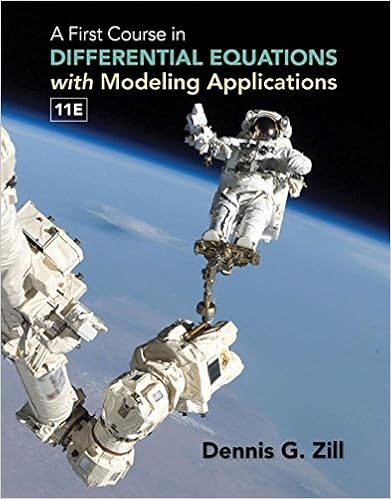# 6 mutation is also a violation of hardy weinberg

• Lab Report
• 3
• 50% (2) 1 out of 2 people found this document helpful

This preview shows page 1 - 3 out of 3 pages.

##### We have textbook solutions for you!
The document you are viewing contains questions related to this textbook.The document you are viewing contains questions related to this textbook.
Chapter 3 / Exercise 1
A First Course in Differential Equations with Modeling Applications
ZillExpert Verified
For a population to stay in Hardy-Weinberg equilibrium, mating must be ___________________. 6.Mutation is also a violation of Hardy-Weinberg conditions, because there can be no _____________________________________________________. 7.Mutations show more quickly in a population. 8.Allelic frequency can also be altered by taxa moving in (termed ___________________) or taxa migrating out (termed ____________________). Either of these can change the composition of the __________________________, also violating Hardy-Weinberg. 9.Another violation of Hardy-Weinberg equilibrium is __________________________, because in a population at equilibrium, no alleles would be selected over any others. 10.To sum up: violations of Hardy Weinberg include a.No mutation b.No migration c.No drift d.Random mating e.No selection
##### We have textbook solutions for you!
The document you are viewing contains questions related to this textbook.The document you are viewing contains questions related to this textbook.
Chapter 3 / Exercise 1
A First Course in Differential Equations with Modeling Applications
ZillExpert Verified
¾Next, read ‘Concept 6’ on ‘Estimating Allelic Frequency’ and answer the question posed there below: Concept 6 question: Can you count the number of recessive alleles in this population? Why or why not? ¾Next, read Concept 7 on ‘The Hardy Weinberg equation’ sample problems for Concepts 8 – 11 below. For each calculation, work the problem on your own paper, but enter your answer here. Concept 8 - Sample Problem 1: 1.) calculate q2 2.) calculate q 3.) calculatep4.) calculate 2pqConcept 9 - Sample Problem 2: How many individuals of fruit flies would you expect to be homozygous for red-colored eyes? Concept 10 - Sample Problem 3 Approximately what percent of the population are heterozygous carriers of the recessive PKU allele?